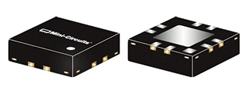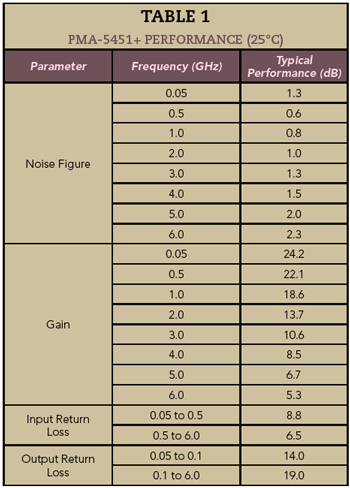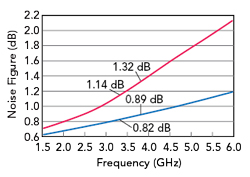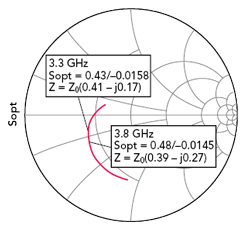www.microwavejournal.com/articles/35791-model-based-optimization-outperforms-lna-datasheet-specifications

# Model-Based Optimization Outperforms LNA Datasheet Specifications

April 14, 2021

It is possible in certain instances to improve the performance of a design that uses a MMIC low noise amplifier (LNA), using the same concepts for discrete transistor LNA designs. In this article, a design using a MMIC LNA is optimized over a 5G frequency band to achieve a lower noise figure than specified on the device datasheet.Figure 1 Single stage LNA topology.

An LNA is a critical component typically found in any receiver chain. Its purpose is to amplify an extremely weak signal captured by the receiver’s antenna, adding minimal noise power to the signal. An LNA’s noise figure is an important parameter enabling a designer to determine whether a given LNA is suitable for a requirement. Noise figure is the decibel representation of noise factor, a measure of the degradation in the signal-to-noise ratio as a signal passes through a network. LNAs are available as MMICs from various manufacturers, and it is often possible to purchase a MMIC LNA with the performance needed for a requirement, rather than having to design an LNA using a discrete transistor. The MMIC LNA can then be incorporated into the overall design by mounting it on a printed circuit board, following the manufacturer’s instructions. In contrast, designing an LNA with a discrete transistor generally requires more effort than using a MMIC because discrete transistor LNA design involves creating appropriate matching networks so the amplifier achieves the desired performance.Figure 2 PMA-5451+ MMIC LNA.

To illustrate, Figure 1 shows a single-stage amplifier design consisting of an input matching network, a transistor and output matching network. The minimum noise figure is achieved when the source reflection coefficient (ΓS) is properly selected; the value of ΓS achieving the minimum noise figure is known as Γopt. Γopt, with the minimum noise figure, Fmin, and the equivalent normalized noise resistance, rn, are provided by the manufacturer of the transistor or can be determined experimentally.1 In general, the goal when designing an LNA with a discrete transistor is to design the input matching network to achieve the lowest noise figure from the transistor and design the output matching network to help the LNA meet the requirements for output return loss, gain, gain flatness and other parameters.1,2

Compared to a discrete transistor LNA design, purchasing a MMIC LNA internally matched to 50 Ω seems easier because there is no need to design any matching networks. Instead, the MMIC is simply inserted into a 50 Ω environment following the manufacturer’s instructions, which is obviously quicker and acceptable in many cases. However, it may be possible to optimize the performance of the MMIC LNA by exploiting the same concepts used for a discrete transistor LNA design. A MMIC LNA may be specified to operate over a wide frequency range, and many designers may assume the noise figure in the datasheet is the best that can be achieved; however, a MMIC LNA may achieve a lower noise figure than shown in the datasheet over a portion of the frequency band. This improved performance can be achieved by optimizing the design over the desired frequency range.

In this article, we optimized an LNA over a 5G band using the PMA-5451+ wideband MMIC LNA from Mini-Circuits. The design was simulated using Keysight Technologies’ Advanced Design System (ADS) software. Modelithics models for the MMIC LNA and all passive components were used, and the Modelithics model for the PMA-5451+ was validated for both S-parameters and noise parameters, which were used to optimize the complete design for the best noise figure over the desired frequency band. The goal was for the noise figure of the optimized LNA to be lower than the typical noise figure for the PMA-5451+ specified in the Mini-Circuits datasheet. Measured data confirmed this approach.

MMIC LNA PERFORMANCE

The Mini-Circuits PMA-5451+ MMIC LNA (see Figure 2) is fabricated using an enhancement-mode PHEMT process. The operating frequency range is specified from 50 MHz to 6 GHz. Operating from a single +3 V DC power supply, the MMIC typically draws 30 mA with a 1.5 kΩ bias resistor. It is assembled in a 3 × 3 mm package. The PMA-5451+ data sheet contains a schematic of the recommended application circuit (see Figure 3) and the characterization test circuit used to determine device specifications. The typical gain, noise figure and input/output return loss at +25°C are shown in Table 1. The table shows the PMA-5451+ has typical noise figures of 1.3 and 1.5 dB at 3 and 4 GHz, respectively. For this design, the goal is to minimize the noise figure from 3.3 to 3.8 GHz, which is the n78 5G NR band.Figure 3 PMA-5451+ application circuit.Figure 4 ADS Modelithics PMA-5451+ model using 20 mil RO4350B substrate.

Modelithics provides models for many Mini-Circuits components, including the PMA-5451+ LNA. The model is a data-based behavioral model developed from broadband S-parameters and noise parameters measured using a Keysight PNA-X vector network analyzer equipped with the ultra-fast noise option. The Modelithics model for the PMA-5451+ is validated for S-parameters and noise parameters at +25°C and biased at +3 V and 30 mA, predicting the S-parameters from 45 MHz to 20 GHz and noise parameters from 500 MHz to 8 GHz—beyond the operating frequency range specified by Mini-Circuits. The model includes three substrate options, since it was extracted from measurements performed using 6.6, 10 and 20 mil thick Rogers RO4350B substrates. Designers can select one of these substrates using the “Substrate” parameter. For this design, the 20 mil thick RO4350B was used. Modelithics provides a datasheet for the PMA-5451+ showing the application schematic used for model extraction. Also included are the model’s S-parameters and noise parameters. The plots from the model datasheet can be replicated by simulating a schematic in ADS containing the PMA-5451+ model with 50 Ω port terminations at the input and output (see Figure 4).

Simulating the schematic of Figure 4, the actual noise figure (NF50 in the model datasheet) and minimum possible noise figure (NFMin in the model datasheet) are plotted in Figure 5, and Figure 6 compares the simulated 50 Ω noise figure with the three substrate thicknesses available in the model. Figure 5 shows the noise figure is 1.14 dB at 3.3 GHz, rising slightly to 1.32 dB at 3.8 GHz. At these same frequencies, the minimum noise figure is 0.82 dB and 0.89 dB, respectively. At 3.3 GHz, the noise figure is 0.32 dB higher than the minimum, with a greater difference of 0.43 dB at 3.8 GHz. By designing a proper matching network, the LNA can be optimized so the noise figure is closer to the minimum values; a realistic goal is a noise figure 1.0 dB from 3.3 to 3.8 GHz.Figure 5 Simulated 50 Ω noise figure (red) and minimum noise figure (blue).Figure 6 Simulated 50 Ω noise figure using 6.6 mil (red), 10 mil (blue) and 20 mil (green) RO4350B substrates.

The optimum source reflection coefficient for the minimum noise figure, known as Sopt in ADS, can also be determined (see Figure 7) and seen in the Modelithics model. To achieve the lowest noise figure, the matching network placed at the input of the PMA-5451+ must produce a source reflection coefficient, ΓS, closely matching Sopt over the frequency range of 3.3 to 3.8 GHz. Figure 8 plots the |S21|, |S11| and |S22| from the same simulation, showing the gain varies between 8.70 and 9.82 dB over the n78 band.Figure 7 Optimum source reflection coefficient for minimum noise figure, 1.5 to 6 GHz.Figure 8 Simulated |S21| (a), |S11| and |S22| (b).

MMIC LNA DESIGN

The ADS schematic for the MMIC LNA design, shown in Figure 9, essentially mirrors Mini-Circuits’ recommended application circuit. The design includes the PMA-5451+, capacitor C1 and inductor L2 located at the input of the MMIC. These two components combined with the microstrip interconnects connecting them to the PMA-5451+ are the input matching network. The capacitor C2 and inductor L1 at the output of the MMIC are selected to achieve acceptable output return loss. The circuit also includes a 1.5 kΩ bias resistor, Rbias, setting the 30 mA bias current, and a bypass capacitor, C3.Figure 9 ADS model of the complete LNA design.Figure 10 Simulated gain (red) and noise figure (blue) of the unoptimized LNA design.

Modelithics Microwave Global Models™ are used for the capacitors, inductors and resistor, which are all 0603 sized parts. AVX SQCS capacitors are used for C1 and C2, Würth Elektronik WE-KI inductors are used for L1 and L2, a KOA Speer RK73H 1.5 kΩ resistor is used for Rbias and a Murata GRM188R72A 0.1 µF capacitor is used for C3. A single Microwave Global Model covers the full range of values for a vendor’s component, and since the values in these models are scalable, the models are useful for tuning or optimizing a design.

To optimize the noise figure from 3.3 to 3.8 GHz, the values of C1 and L2 must be suitably adjusted. As the lengths of the microstrip interconnects connecting C1 and L2 to the PMA-5451+ play a role in achieving the best performance, the optimization process includes tuning the lengths of the microstrip interconnects, as well as adjusting the component values. To establish a starting point for the design, the gain and noise figure of the LNA were simulated before optimizing the component values and interconnect dimensions, by setting C1 and L2 to the initial values of 100 pF and 390 nH, respectively, and the microstrip interconnect lengths to arbitrary values. At 3.8 GHz, the starting gain was just under 8 dB and the noise figure slightly greater than 1.6 dB (see Figure 10).Figure 11 Discrete elements optimized with the Modelithics passive component model parametersThe next step was optimizing the design to reduce the noise figure, a process made simpler using the “discrete optimization” feature within the Microwave Global Models (see Figure 11). This feature makes it possible to perform discrete optimization, where the model’s part value is adjusted to the manufacturer’s “real life” part value.3 With this method, designers can indicate the range of part values within a part family to be included in the optimization. In this case, the discrete optimization feature was activated for C1 and L2, the components at the input of the PMA-5451+, to achieve the best noise figure. The optimization goal must be specified and the optimization itself configured. An optimization goal of 0.9 dB maximum noise figure - probably unrealistic - was set over the range from 3.3 to 3.8 GHz, and a discrete optimization performed, combined with tuning the microstrip interconnect lengths, to obtain the best noise figure over the n78 band. In addition, the values of the components at the output of the PMA-5451+, C2 and L1, were determined to achieve adequate output return loss. After optimization, the values were C1 = 2 pF, L2 = 27 nH, C2 = 3.6 pF and L1 = 3.3 nH (see Table 2).Figure 12 Simulated noise figure of the LNA before (dotted) and after (red) optimization.Figure 13 Simulated |S21| before (dashed black) and after (red) optimization, |S11| (blue) and |S22| (magenta).

Figure 12 shows the simulated noise figure of the optimized LNA design, comparing it to the simulated noise figure before optimization (from Figure 10). After optimization, the simulated noise figure was < 1.0 dB from 3.3 to 3.8 GHz. Figure 13 shows the simulated gain and return loss of the optimized LNA design. The stability factor was > 1 over the entire simulated frequency range from 50 MHz to 8 GHz. Figure 14 compares the Sopt data of the PMA-5451+ model to the reflection coefficient looking into the input matching network from the PMA-5451+. To achieve optimal noise figure from 3.3 to 3.8 GHz, the source reflection coefficient should be as close as possible to Sopt, which is shown in the figure.Figure 14 PMA-5451+ Sopt (blue circles) and reflection coefficient looking into the input matching network from the PMA-5451+ (red).Figure 15 LNA assembly.

LNA MEASUREMENTS

The final step was validating the design by building and measuring the LNA; Figure 15 shows one of two units that were assembled and measured. The measured gain and return loss largely agree with the simulations (see Figures 16 and 17). Figure 18 compares the measured and simulated noise figure, including the simulated noise figure before optimization. One of the two measured LNAs had a noise figure < 1 dB from 3.3 to 3.8 GHz, while the noise figure of the second LNA was slightly higher, peaking at 1.06 dB at 3.8 GHz. We believe assembly variability and measurement sensitivity caused the higher noise figure and deviation from the simulation. Nonetheless, the results confirm that reducing the noise figure was achieved.Figure 16 Measured gain of two LNAs (dashed) vs. simulated gain (red).Figure 17 Measured vs. simulated |S11| (a) and |S22| (b). The dashed traces represent measurements of two LNAs; the red traces are simulated performance.

CONCLUSIONFigure 18 Measured noise figure of two LNAs (dashed) vs. simulation before (dotted black) and after (red) optimization.

The test results demonstrate a MMIC LNA can be optimized to achieve lower noise figure than specified in the manufacturer’s data sheet over a narrower frequency range. Designers can use this approach to achieve lower noise figure than specified for a MMIC. Modelithics amplifier models are well-suited for this approach because they enable designers to predict both S-parameters and noise parameters. Adding the ability to scale the model values of passive components makes it easy to optimize the passive elements to meet design goals.

References

1. Guillermo Gonzalez, Microwave Transistor Amplifiers: Analysis and Design, Second Edition, Prentice Hall, New Jersey, 1997.
2. S. Akamatsu, S. Muir and L. Dunleavy, “Simulation Procedures for Successful Low Noise Amplifier (LNA) Design Using Discrete Components,” High Frequency Electronics, 2012, pp. 2230.
3. “Discrete Optimization with Modelithics Models in ADS,” Modelithics, October 2018, Web, www.modelithics.com/Literature/Presentation.